# Worked Example: Species Distribution

This vignette demonstrates acquiring, processing and rudimentarily visualising the distribution of Colibri species.

## Acquire data

The query uses the quality = "research" parameter to focus on more reliable data (i.e. observations that have been identified as the same taxon by several iNaturalist contributors, of animals that are not in captivity).

library(rinat)
colibri <- get_inat_obs(taxon_name = "Colibri",
quality = "research",
maxresults = 1000)

Which species are listed?

library(dplyr)
colibri %>% pull(scientific_name) %>% unique()
#>  "Colibri cyanotus"            "Colibri coruscans"           "Colibri cyanotus cabanidis"
#>  "Colibri delphinae"           "Colibri thalassinus"         "Colibri serrirostris"
#>  "Colibri coruscans coruscans"

## Prepare the data

We can process the data to merge the subspecies into the corresponding species, and remove rows with missing coordinates. Parsing the date and time of observation can also be useful.

library(stringr)
library(lubridate)
col_sp <- colibri %>%
filter(!is.na(longitude)) %>%
mutate(scientific_name = word(scientific_name, 1, 2)) %>%
mutate(datetime = ymd_hms(datetime))

## Visualise

A histogram can be useful to get an idea of the range of dates.

library(ggplot2)
ggplot(col_sp, aes(x = datetime)) +
geom_histogram()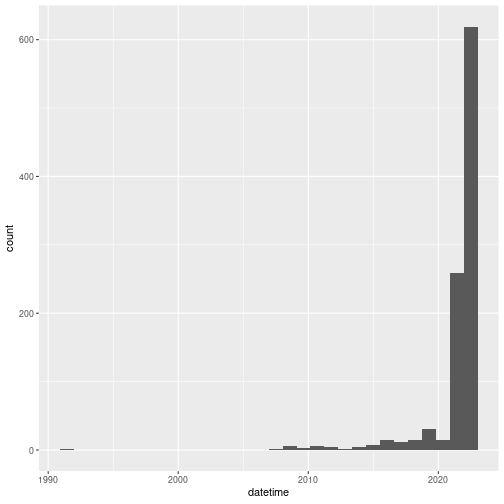plot of chunk date_hist

Expectedly, observations are mostly recent. Although earlier observations can be submitted, iNaturalist was launched in 2008, which means the vast majority of the observations are from after 2008.

Let’s now visualise the observations’ locations with ggplot2.

ggplot(data = col_sp, aes(x = longitude,
y = latitude,
colour = scientific_name)) +
geom_polygon(data = map_data("world"),
aes(x = long, y = lat, group = group),
fill = "grey95",
color = "gray40",
size = 0.1) +
geom_point(size = 0.7, alpha = 0.5) +
# this guarantees the map is not stretched
coord_fixed(xlim = range(col_sp$longitude), ylim = range(col_sp$latitude)) +
theme_bw()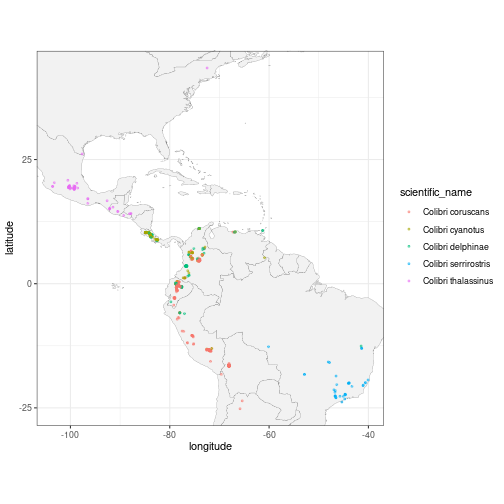plot of chunk viz_col

Using ggforce’s geom_mark_*() functions, we can highlight and annotate the different species directly on the plot. We expand the x and y axes limits to make space for the additions.

# define expansion factors for giving space to ellipses and labels
exp_fact <- c(0.5, 0.5)
library(ggforce)
ggplot(data = col_sp, aes(x = longitude,
y = latitude,
colour = scientific_name)) +
geom_polygon(data = map_data("world"),
aes(x = long, y = lat, group = group),
fill = "grey95",
color = "gray40",
size = 0.1) +
geom_point(size = 0.7, alpha = 0.5) +
# annotate with a hulls and labels
geom_mark_hull(aes(label = scientific_name,
fill = scientific_name),
expand = unit(1, "mm"),
label.fill = "transparent") +
# expand axes here
scale_x_continuous(expand = exp_fact) +
scale_y_continuous(expand = exp_fact) +
coord_fixed(xlim = range(col_sp$longitude), ylim = range(col_sp$latitude)) +
theme_bw() +
# remove the superfluous legend
theme(legend.position = "none")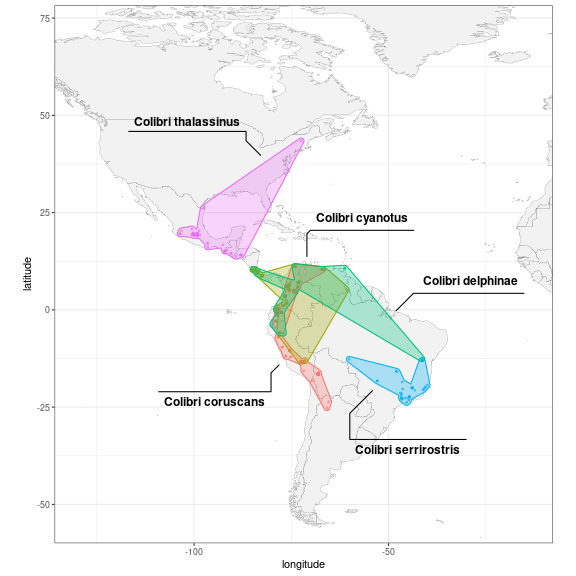plot of chunk viz_col_annotated

## Remove outliers

Some observations could have been misidentified, misplaced, or simply be an exception that is not representative of the common range of the species. One way to remove these outliers could be to compute for each observation the distance to the nearest other observation belonging to the same species. For that, we first convert to an sf object, split it into separate species, and use the functions st_nearest_feature() to identify the closest feature and st_distance() to compute the distance.

library(sf)
library(purrr)
col_dist <- col_sp %>%
st_as_sf(coords = c("longitude", "latitude"), crs = "WGS84") %>%
split(.$scientific_name) %>% map_dfr(~ mutate(.x, nearest_id = st_nearest_feature(.x), nearest = geometry[nearest_id], dist_nearest = st_distance(geometry, nearest, by_element = TRUE))) Let’s highlight the observations that are at least 1000 km away from another observation of the same species: ggplot(data = col_dist, aes(colour = scientific_name)) + geom_sf() + geom_sf(data = col_dist %>% filter(as.integer(dist_nearest) > 1e6), colour = "red", size = 5, shape = 1)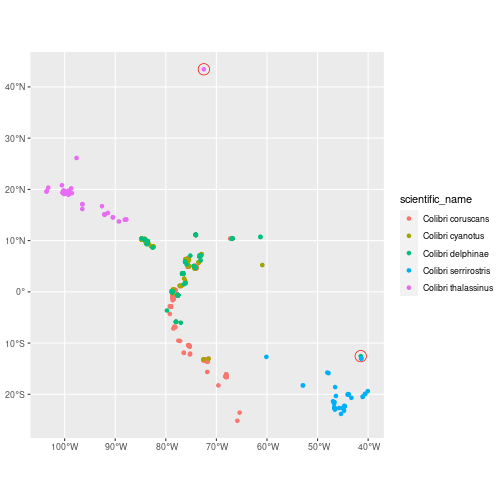plot of chunk show_distant We can now reuse the same visualisation code as before, with the outliers filtered out: col_clean <- col_sp %>% anti_join(col_dist %>% filter(as.integer(dist_nearest) > 1e6), by = "id") # filter outliers out ggplot(col_clean, aes(x = longitude, y = latitude, colour = scientific_name)) + geom_polygon(data = map_data("world"), aes(x = long, y = lat, group = group), fill = "grey95", color = "gray40", size = 0.1) + geom_point(size = 0.7, alpha = 0.5) + geom_mark_hull(aes(label = scientific_name, fill = scientific_name), expand = unit(1, "mm"), radius = unit(1, "mm"), label.fill = "transparent") + scale_x_continuous(expand = exp_fact) + scale_y_continuous(expand = exp_fact) + coord_fixed(xlim = range(col_clean$longitude),
ylim = range(col_clean\$latitude)) +
theme_bw() +
theme(legend.position = "none")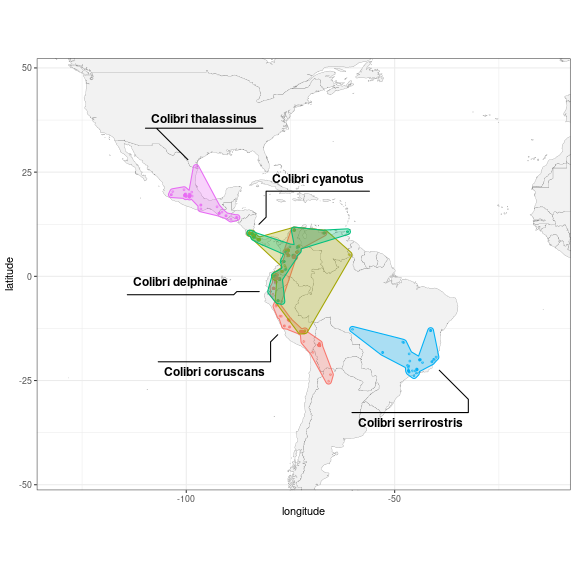plot of chunk viz_col_clean

Note that this outlier detection method will not always be useful. For example, one outlier specimen could have been recorded in several separate observations, and therefore wouldn’t be filtered out based on distance.